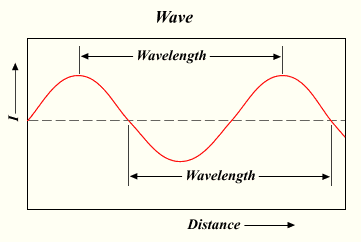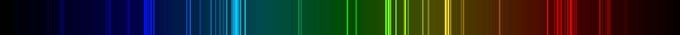## Planck’s Quantum Theory

#### Learning Objective

• Calculate the energy element E=hv, using Planck’s Quantum Theory

#### Key Points

• Until the late 19th century, Newtonian physics dominated the scientific worldview. However, by the early 20th century, physicists discovered that the laws of classical mechanics do not apply at the atomic scale.
• The photoelectric effect could not be rationalized based on existing theories of light, as an increase in the intensity of light did not lead to the same outcome as an increase in the energy of the light.
• Planck postulated that the energy of light is proportional to the frequency, and the constant that relates them is known as Planck’s constant (h). His work led to Albert Einstein determining that light exists in discrete quanta of energy, or photons.

#### Terms

• photoelectric effectThe emission of electrons from the surface of a material following the absorption of electromagnetic radiation.
• electromagnetic radiationRadiation (quantized as photons) consisting of oscillating electric and magnetic fields oriented perpendicularly to each other, moving through space.

In the late 18th century, great progress in physics had been made. Classical Newtonian physics at the time was widely accepted in the scientific community for its ability to accurately explain and predict many phenomena. However, by the early 20th century, physicists discovered that the laws of classical mechanics are not applicable at the atomic scale, and experiments such as the photoelectric effect completely contradicted the laws of classical physics. As a result of these observations, physicists articulated a set of theories now known as quantum mechanics. In some ways, quantum mechanics completely changed the way physicists viewed the universe, and it also marked the end of the idea of a clockwork universe (the idea that universe was predictable).

Electromagnetic (EM) radiation is a form of energy with both wave-like and particle-like properties; visible light being a well-known example. From the wave perspective, all forms of EM radiation may be described in terms of their wavelength and frequency. Wavelength is the distance from one wave peak to the next, which can be measured in meters. Frequency is the number of waves that pass by a given point each second. While the wavelength and frequency of EM radiation may vary, its speed in a vacuum remains constant at 3.0 x 108 m/sec, the speed of light. The wavelength or frequency of any specific occurrence of EM radiation determine its position on the electromagnetic spectrum and can be calculated from the following equation:

$c=\lambda\nu$

where c is the constant 3.0 x 108 m/sec (the speed of light in a vacuum), $\lambda$ = wavelength in meters, and $\nu$=frequency in hertz (1/s). It is important to note that by using this equation, one can determine the wavelength of light from a given frequency and vice versa.Wavelength of EM radiationThe distance used to determine the wavelength is shown. Light has many properties associated with its wave nature, and the wavelength in part determines these properties.

## The Discovery of the Quantum

The wave model cannot account for something known as the photoelectric effect. This effect is observed when light focused on certain metals emits electrons. For each metal, there is a minimum threshold frequency of EM radiation at which the effect will occur. Replacement of light with twice the intensity and half the frequency will not produce the same outcome, contrary to what would be expected if light acted strictly as a wave. In that case, the effect of light would be cumulative—the light should add up, little by little, until it caused electrons to be emitted. Instead, there is a clear-cut minimum frequency of light that triggers electron ejection. The implication was that frequency is directly proportional to energy, with the higher light frequencies having more energy. This observation led to the discovery of the minimum amount of energy that could be gained or lost by an atom. Max Planck named this minimum amount the “quantum,” plural “quanta,” meaning “how much.” One photon of light carries exactly one quantum of energy.

Planck is considered the father of the Quantum Theory. According to Planck: E=h$\nu$, where h is Planck’s constant (6.62606957(29) x 10-34 J s), ν is the frequency, and E is energy of an electromagnetic wave. Planck (cautiously) insisted that this was simply an aspect of the processes of absorption and emission of radiation and had nothing to do with the physical reality of the radiation itself. However, in 1905, Albert Einstein reinterpreted Planck’s quantum hypothesis and used it to explain the photoelectric effect, in which shining light on certain materials can eject electrons from the material.

## More Evidence for a Particle Theory of Energy

When an electric current is passed through a gas, some of the electrons in the gas molecules move from their ground energy state to an excited state that is further away from their nuclei. When the electrons return to the ground state, they emit energy of various wavelengths. A prism can be used to separate the wavelengths, making them easy to identify. If light acted only as a wave, then there should be a continuous rainbow created by the prism. Instead, there are discrete lines created by different wavelengths. This is because electrons release specific wavelengths of light when moving from an excited state to the ground state.Emission spectrum of nitrogen gasEach wavelength of light emitted (each colored line) corresponds to a transition of an electron from one energy level to another, releasing a quantum of light with defined energy (color).